Courses

# NCERT Exemplar - Areas Related to Circles Class 10 Notes | EduRev

## Class 10 : NCERT Exemplar - Areas Related to Circles Class 10 Notes | EduRev

The document NCERT Exemplar - Areas Related to Circles Class 10 Notes | EduRev is a part of the Class 10 Course Mathematics (Maths) Class 10.
All you need of Class 10 at this link: Class 10

Q.1. All the vertices of a rhombus lie on a circle. Find the area of the rhombus, if area of the circle is 1256 cm2 (Use π = 3.14).
Ans.
Area of the circle = 1256 cm2
⇒ A = πr2
A.T.Q. 1256 = πr2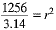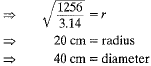Here, Diagonals of rhombus = diameter of the circle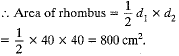Q.2. Find the number of revolutions made by a circular wheel of area 1.54 m2 in rolling a distance of 176 m.
Ans.
Area of wheel = 1.54 m2
A = πr2
1.54 = πr2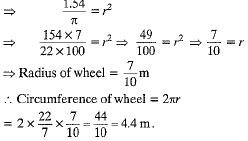Distance covered in one revolution = 4.4 m
No. of revolutions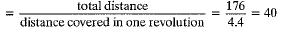Q.3. An archery target has three regions formed by three concentric circles as shown in Fig. If the diameters of the concentric circles are in the ratio 1 ; 2 : 3, then find the ratio of the areas of three regions.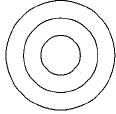Ans.
Let, diameter of inner most circle = x
Diameter of middle circle = 2x
Diameter of outer most circle = 3x
∴ Area of inner most circle = π(x)2
Area of middle circle =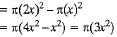Area of outer most circle =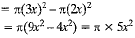Ratio of the areas of three regions.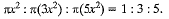Offer running on EduRev: Apply code STAYHOME200 to get INR 200 off on our premium plan EduRev Infinity!

## Mathematics (Maths) Class 10

59 videos|362 docs|103 tests

,

,

,

,

,

,

,

,

,

,

,

,

,

,

,

,

,

,

,

,

,

;